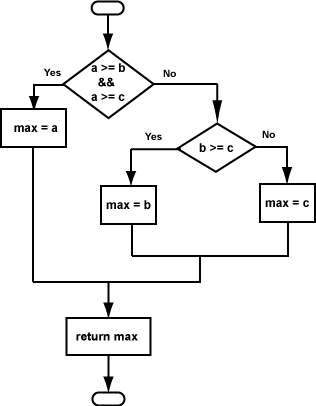Two if-structures should do the trick

# Structured Max ThreeThe flowchart shows this function. The code for this is

```
/*
pre-condition: a, b, and c are integers.
exit condition: return the maximum of a, b, and c
*/

int maxThree(int a, int b, int c)
{
int max;
if (a >= b && a >= c)
max = a;
else
if (b >= c)
max = b;
else
max = c;

return max;
}
```

The flowchart is easy to follow, and the code matches it, so this is a good structured design.

### QUESTION 2:

If the maximum is `a`, how soon in the code is this known?